News & Events

# How to Find Gear Modification Coefficient

How to calculate gear modification coefficient:
1. Total displacement coefficient: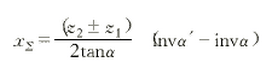Total displacement coefficient

2. Coefficient of variation of center distance: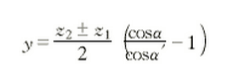Coefficient of variation of center distance

3. Coefficient of change of addendum height: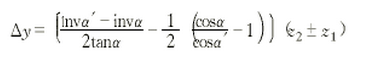Coefficient of change of addendum height

4. The modification coefficient table of the number of teeth z=8～20 cylindrical gear: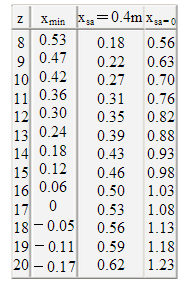The modification coefficient table of the number of teeth z=8～20 cylindrical gear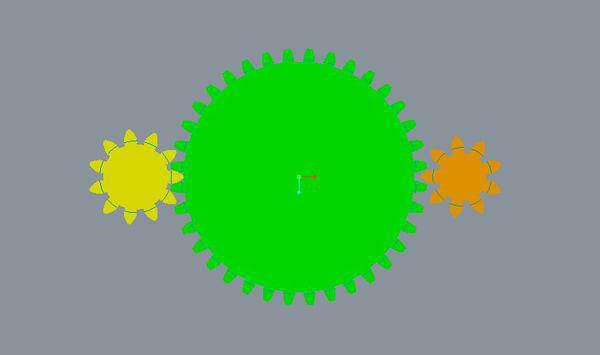Gear Modification Coefficient